# How to use the COUNTIFS function in Excel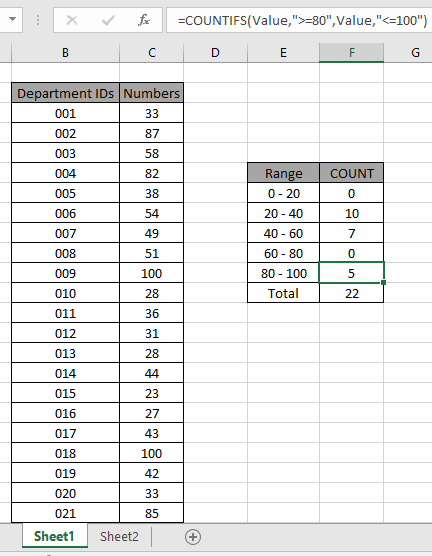In this article, we will learnHow to use the COUNTIFS function in Excel.

What is the COUNTIFS function ?

Many times when working with data where we need to find the numerical count of values having criteria. For example finding the number of employees having salaries between 1 million to 10 million dollars. As you can notice this function can take multiple criteria in one formula. Let's learn this function syntax and an example to illustrate its use.

COUNTIFS Function in Excel

Syntax :

 =COUNTIFS(criteria_range1, criteria1, criteria_range2, criteria2)

Criteria_range1: This is the first criteria range. This is mandatory. Your first condition will be from this range.

Criteria1: This is the first criteria from criteria range1.

Criteria_range2: This is the second criteria range for your second condition. This can be the same as the first one.

Criteria2: The criteria2 is the second condition from criteria range 2.

Example :

All of these might be confusing to understand. Let's

understand how to use the function using an example.

Here we have the ID records and we need to find the COUNT of ID values where respective numbers lays between the given range.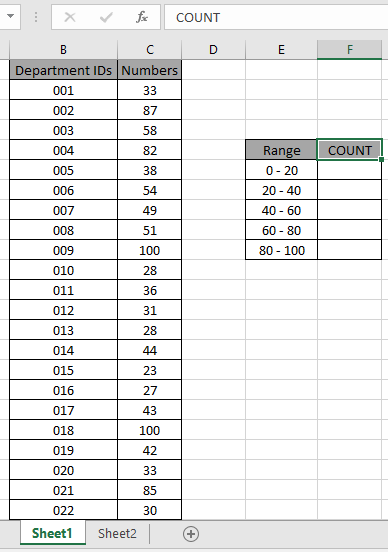Here to find the values in range. Some range references given using named range.

Numbers array given as Value named range for the array C3:C24.

Use the formula:

 = COUNTIFS ( Value , ">=0" , Value , "<20" )

Explanation:

• COUNTIFS function counts the cells that match the numbers in the Value array which are greater than equals to 0 (0 included).
• COUNTIFS function counts the cells that match the numbers in the Value array which are less than 20 (20 excluded).
• The formula counts the cell which satisfies both conditions which means value which lay between 0 to 20 will be counted.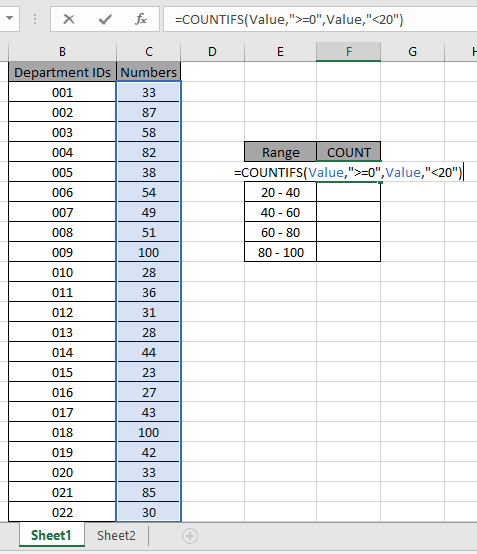The formula looks like as shown in the above snapshot. The Value array is given as a named range.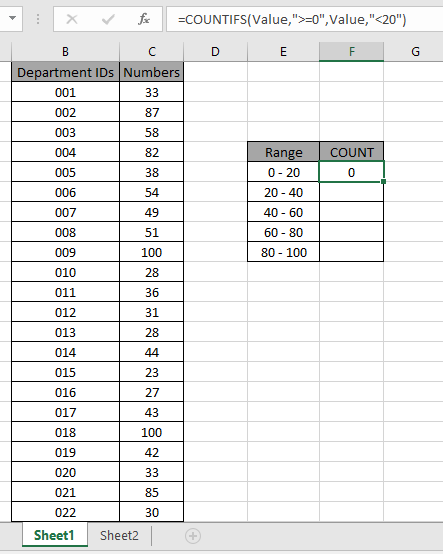As you can see the total values which occur between 0 - 20 comes out to be 0 i.e. there are no such values between 0 - 20.

Now copy the formula in other cells using the drag down option or using the shortcut key Ctrl + D as shown below. And changing the range parameter to 20 - 40.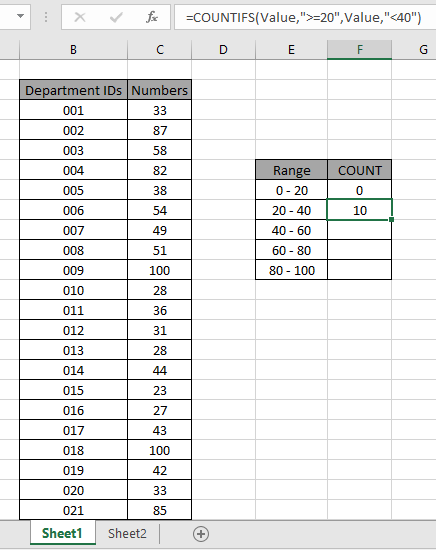As you can see values which lay between 20 - 40 are 10.

Now calculate all the other counts with given range values as explained above.We obtained all the counts between given range as it also proves the formula works fine.

Another Example:

We have this data for our next COUNTIFS example.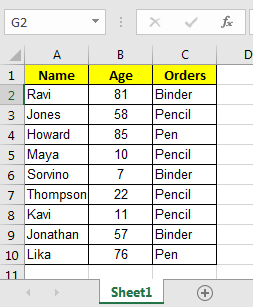And, we have these two queries to answer.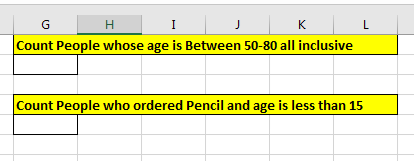So for the first query we have two conditions from one single column, Age.

To answer the query, Count People whose age is Between 50-80 all inclusive, write this COUNTIFS formula.

 =COUNTIFS(B2:B10,">=50",B2:B10,"<=80")

Note that, we have the same criteria range B2:B10  for both conditions. Excel has no objection to the use of the same criteria ranges. With the picked data output will be 3.

How it Works?

Simple, the k function will first look for all values in range B2:B10 that will be {81,58,85,57,76}. Then in the {81,58,85,57,76} list it will look at all values that are less than or equal to 80 and that will be {58,57,76}. And that is 3.

Now in the second query in which I need to Count People who ordered Pencil and age is less than 15. We have to count on multiple criteria and different columns.

So  two answers to this query write this COUNTIFS formula.

 =COUNTIFS(C2:C10,"Pencil",B2:B10,"<15")

Note that we used two different columns for our condition.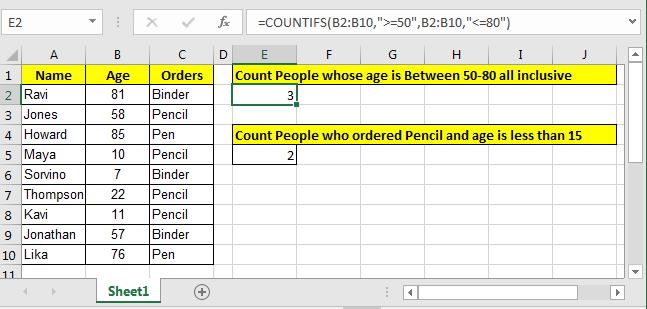Well this is the  classic way of counting in excel for two or more criteria. But there is an alternative way to count if two criteria match.

You can also use the alternative function inplace of the COUNTIFS function. Learn How to use the SUMPRODUCT function in Excel

Here are some observational notes shown below.

Notes:

1. The formula only works with numbers and text both.
2. The formula ignores text value while comparing numbers and ignores numbers when match text values.
3. Operators like equals to ( = ), less than equal to ( <= ), greater than ( > ) or not equals to ( <> ) can be performed within a function applied with number only.

Hope this article about How to use the COUNTIFS function in Excel is explanatory. Find more articles on calculating values and related Excel formulas here. If you liked our blogs, share it with your friends on Facebook. And also you can follow us on Twitter and Facebook. We would love to hear from you, do let us know how we can improve, complement or innovate our work and make it better for you. Write to us at info@exceltip.com.

Related Articles :

COUNTIFS with Dynamic Criteria Range : Count cells dependent on other cell values in Excel.

COUNTIFS Two Criteria Match : Count cells matching two different criteria on list in excel.

COUNTIFS With OR For Multiple Criteria : Count cells having multiple criteria match using the OR function.

How to Use Countif in VBA in Microsoft Excel : Count cells using Visual Basic for Applications code.

How to use the SUMPRODUCT function in Excel: Returns the SUM after multiplication of values in multiple arrays in excel.

How to use wildcards in excel : Count cells matching phrases using the wildcards in excel

Popular Articles :

How to use the IF Function in Excel : The IF statement in Excel checks the condition and returns a specific value if the condition is TRUE or returns another specific value if FALSE.

How to use the VLOOKUP Function in Excel : This is one of the most used and popular functions of excel that is used to lookup value from different ranges and sheets.

How to use the SUMIF Function in Excel : This is another dashboard essential function. This helps you sum up values on specific conditions.

How to use the COUNTIF Function in Excel : Count values with conditions using this amazing function. You don't need to filter your data to count specific values. Countif function is essential to prepare your dashboard.

Terms and Conditions of use

The applications/code on this site are distributed as is and without warranties or liability. In no event shall the owner of the copyrights, or the authors of the applications/code be liable for any loss of profit, any problems or any damage resulting from the use or evaluation of the applications/code.